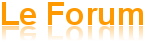ANALYSIS Forum (IPACO)
Dedicated to the analysis of alleged UFO photos and videos

Author Message
Haraka
New member II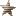Offline

Joined: 21 Aug 2012
Posts: 12
Localisation: TanzaniaPosted: 04/08/2015, 11:30 am    Post subject: Continuing on the Topic of Imagery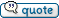Outlining  Some Imagery Enhancement  Principles and Introducing the Spatial Domain (ii)                   In the first part of this article we looked at the concept of resolution from principally the radiometric  (or  “gray level”)  aspect  of imagery. It was demonstrated how this could be made more interpretable by enhancing information contained within the image that was not normally perceivable by the observer.           The concept of resolution in imagery interpretation is multi–dimensional. The  axes of resolution of  imagery  also spread across the spectral domain ( “color”)  , the temporal domain  (the time  interval(s)  between images being captured) as well as the spatial ( “detail”)  domain which we introduced at the end of  (i) .  Incidentally this last domain is often commonly and incorrectly  touted  as “ the  resolution”  of  imagery,  in disregard of  the other three imagery resolution domains.            The important concept of spatial frequency outlined in (i) enables us to address characteristics contained within the spatial domain of an image. To recap: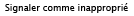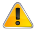Fig. 1.Low Spatial Frequency Component  (a) across “x” axis of image e.g. cloud (b)                  This representation of a spatial frequency component shows a typical low frequency sine wave typified by gradual changes across the image, such as with overcast cloud.          The sine wave pattern shown above can be expressed in a simple concept  that involves three possible variables, The spatial frequency, the amplitude (positive or negative), and the phase.Fig.2 . High Spatial Frequency Component  (a) across “x” axis of  image  e.g. pebbles (b).           In contrast this spatial frequency component typifies a more densely packed variation in radiometry across the image .The representation of an image in terms of all the spatial frequencies contained within it brings  in a branch of mathematics  that  enables us to transform the representation of an image from the normally perceived  spatial domain  ( i.e. “picture”)  across to the frequency domain. The formula by which we convert, or transform, an image from one domain to the other is referred to as a domain transform and the most commonly used of these is the Fourier Transform .        Joseph Fourier (1768-1830) was a French physicist who developed a range of functions , based upon frequency, which are  used in  many branches of mathematics and science.  In digital imagery the image can be seen as a function of two variables ( the pixel value is the function, its  “x” and “y”  co-ordinates  the two variables) .The Fourier transform can  display a new representation of an image, based upon the spatial frequency components, and preserving all the information in the original image.  Complicated functions can be represented in this way and worked upon in an easier manner.        We can represent these frequencies graphically by re displaying the image information in terms of the spatial frequencies contained within it in the frequency domain , rather than as a pixel matrix in the spatial domain..           So, for our first case above, the low spatial frequency  across the image  can be represented as seen below:Fig.3.  Low spatial frequency (a) across image “x” axis expressed as a frequency component (b).           Note that the  center point –the origin ( which actually represents  the mean brightness level or  signal amplitude in  the image)  is bracketed by two points close in, i.e. indicating  a low spatial frequency  . This pair of points represents a particular spatial frequency  in the image along the “x” axis. Also note that these two points are symmetrical in distance from the origin. This opposing symmetry of frequencies always appears in an image expressed in spatial frequency terms.          It is perhaps helpful to note at this point that this is not just abstract theory. If a lens has  a slide  of  Fig. 3(a)  above  placed at its focal length and a piece of frosted glass put at its  focal plane then a beam of coherent ( i.e. laser) light shone through the lens will produce an image identical to that at Fig. 3 (b) above on the glass. The lens is actually performing the transform operation optically.Fig. 4. Higher  Spatial frequency ( a) in “x” axis i.e. across image , expressed as a frequency component ( b).          Do note that in Fig.4. above the two points are more displaced outwards towards the left and right  edges then in Fig. 3.,being representative of a higher spatial frequency.       So far we have only  looked across the image. If we now rotate 90 deg. and look  up and down the image’s spatial frequencies, precisely the same transformation occurs in the  “y” axis , we just have to rotate everything  accordingly  thus:Fig. 5. Spatial Frequency in “y”  axis i.e. up and down  image (a)  expressed as a frequency component ( b).Fig. 6. The same rules apply through 360 degrees in the plane of the image .              So, if we add all the of  spatial frequencies in all  orientations in the x,y,  plane to get the Fourier transform of an image , we typically end up with an expression of the image in the frequency domain as looking  something like this:.Fig.7. Typical Fourier Transform of an image.            This representation of an image in the frequency domain contains all the original  spatial information.  Indeed if the Fourier transform is inverted ( i.e. “reversed” ) the original image will be faithfully reconstructed in the spatial domain . The original picture information is all intact. Note the brightness along the  “x” ( horizontal ) and “y” ( vertical axes)   these indicate an image with distinct  vertical and horizontal features, such as one might see in doors  or windows for  example.         In the transform the low spatial frequencies are towards the central origin  and the higher spatial frequencies ranging  out to periphery. So if ,for example, we wish to reduce high spatial frequency “noise” we can remove the outer parts of this frequency domain transform before running the inverse transform. Thus an image can be “smoothed”. As we are letting the low spatial frequencies dominate in the image,  this  process is often referred to as “low-pass”  filtering.Fig 8. ” Low Pass”  Fourier filter.                                      Conversely removing lower frequencies close to the  center  will “sharpen” the image for the observer.  (a.k.a.” High Pass”  Filtering)Fig.9. “High Pass” Fourier  filter.            So, to recap, the  various  changes in radiometry  across the image in all directions are open to be  detected and measured  in terms of spatial frequency . In a manner conceptually somewhat analogous to that used to group pixels by their radiometry (as outlined in the earlier paper)  instead of  their physical  positions  (x,y,) in an image.      The spatial frequency components of an image can be re-plotted from low to high  on new “x” and “y”  axes across the frequency range. Although looking nothing like a “picture” all the information in the original image  is retained and the image can be reconstructed back in to the spatial domain by reversing the process. The varying spatial frequencies can be attenuated in order to make the image more interpretable , however  no additional external  information is added to the image.            However it is not necessary to always move an image from the spatial domain to the frequency domain in order to perform filtering operations . The image can be directly and specifically filtered in the spatial domain by a process known as image convolution. This operation, in essence, looks at the relationship of a pixel to those pixels around  it. It is often referred to as a neighborhood operation.          In this routine each pixel is systematically looked at in turn and its relationship with the pixels around it weighted. The end result being an image that can be high pass, low pass or edge enhanced in a number of ways.  The  mathematical instrument that looks at and adjusts this relationship is called a convolution kernel or, alternatively, a “mask” in some texts.            Here is a schematic diagram of a typical convolution kernel or “mask” .Fig.10.  A typical convolution kernel.                 Several points can be noted. This kernel covers a 9 element matrix of 3x3 pixels centered on the pixel  being weighted  at value 16 in this example – which is of a typical high pass filter incidentally.   Many convolution kernels are of this size for a number of reasons, primarily because the small size is easier to implement compared to bigger kernels,  e.g. 7x7, 9x9 up to  25x25 or more  for some specialist  low-pass operations that generate exponentially greater number of calculations: hence the practicability of working in the Fourier domain for some complex tasks.  However,  some of the bigger sizes can be  approximated by  performing a series of 3x3 convolutions.    The convolution  kernel scans the image from top left , pixel by pixel, row by row as can be conceptually visualized below.Fig 11. Schematic of Convolution Kernel Scanning an image Pixel Matrix.                    The convolution kernel addresses each image pixel value in turn as it reads across the rows and down the columns of  the  image.  Above we can see that is centered on a pixel with a gray level value of “8”. We’re using single digit figures here  to keep the math in check, incidentally.               The input value for the pixel is “8”. Now each of the nine pixels covered in the  original matrix  is first of all multiplied by the weighting value in its corresponding  position in the kernel . The top left  pixel in the grayed out section of the image has the value 8. This is multiplied by the top left value in the kernel which is  “-1” .So  (-1 ) x 8  = -8 .               The kernel then adds all of these values up for the nine pixels covered  as shown schematically below:                                   (-1x8) + (-1x2) + ( -1x2)                                                  +                                 (-1x6) + (16x8) + (-1 x2)                                                  +                                 (-1x6) + (-1x6) + (-1x8)                The sum total of all these values is (128- 40 )  i.e.     88             This  kernel then divides this figure by the sum of all the values contained  within the kernel itself  i.e.( 16-8)  = 8                                              88/8  = 11            “11”  is then inserted in to the output image in the place of the original pixel value  of  “8” and the kernel moves on to the next pixel in the original image and repeats the process. .            This is a typical example of a high frequency pass kernel, in which relatively lower values become lower whilst the higher values become higher. A kernel with these characteristics reversed will act as a low pass or “smoothing” filter.              It might be helpful now to look at a couple of image enhancements in order to illustrate these operations in practice .                We can start by looking at a copy of one of the most famous “ UFO” images of the ‘50’s ( and the very first one seen by the author at a tender age).Fig.11. George Adamski “ Flying Saucer”.                  If this image is high pass filtered by a convolution  kernel similar to the one used in the example above, a more “crisp“ image emerges.Fig 12 .Original Adamski Image (a). High pass filtered (b).               Note, however, that although the rim  of the  saucer and the edges of the apparent windows appear sharper  the “noise” in the image is also more apparent, for example in the  background area towards the lower left of the picture.     This process is somewhat analogous to adjusting the bass and treble output  on a music  sound system. The desired result can be heard, but all the  original information is still there in the  recording.               A typical edge enhancement convolution kernel can bring out features as  illustrated  below:Fig 13 .Original Adamski  Image (a). Edge enhancement  filtered ( b)               In practice the edge enhancement routine is usually added back in to the original image in many cases to improve its appearance for  many applications, ranging from imaging satellites to personal digital cameras.             In a future article we will look at some practical examples of the application of imagery enhancement techniques in UFO imagery.PublicitéPosted: 04/08/2015, 11:30 am    Post subject: Publicité

 Publicité Supprimer les publicités ?Display posts from previous: All Posts1 Day7 Days2 Weeks1 Month3 Months6 Months1 Year Oldest FirstNewest First
 All times are GMT + 2 Hours Page 1 of 1

 Jump to: Select a forum----------------Board announcements & news   New members introduction   FAQ - Forum rules   Updates - Informations   SaloonAnalysis: photos and Videos   Class definitions   Photos - IFO (class "A" and "B")   Photos - UFO (class "C")   Photos - UFO (class "D1" and "D2")   Videos - IFO (class "A" and "B")   Videos - UFO (class "C")   Videos - UFO (class "D1" and "D2")   New cases: submissions and discussions   Old cases - DiscussionAnalysis: technical subjects   Articles and publications   Softwares, IPACO and others   Tools kit   Geoff's columnOptics in photography   Overview   Aberrations and effects

Index | Create free forum | Free support forum | Free forums directory | Report a violation | Conditions générales d'utilisation
Powered by phpBB © 2001, 2005 phpBB Group